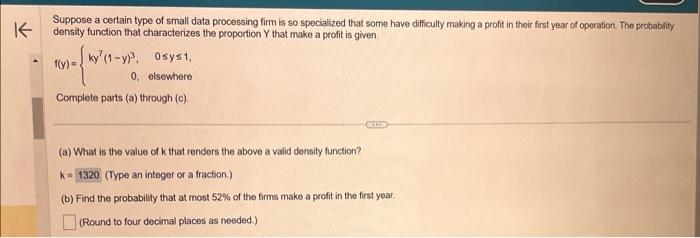Home / Expert Answers / Statistics and Probability / part-b-suppose-a-certain-type-of-small-data-processing-firm-is-so-specialized-that-some-have-difficu-pa228

# (Solved): part b Suppose a certain type of small data processing firm is so specialized that some have difficu ...

part bSuppose a certain type of small data processing firm is so specialized that some have difficulty making a profit in thair first year of operation. The probablity density function that characterizes the proportion $$Y$$ that make a profit is given. $f(y)=\left\{\begin{array}{rr} k y^{7}(1-y)^{3}, & 0 \leq y \leq 1 \\ 0, & \text { etsewhere } \end{array}\right.$ Complete parts (a) through (c). (a) What is the value of $$k$$ that renders the above a valid density function? $$k=\quad$$ (Type an integer or a fraction.) (b) Find the probability that at most $$52 \%$$ of the firms make a profit in the first year. (Round to four decimal places as needed.)

We have an Answer from Expert